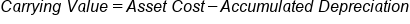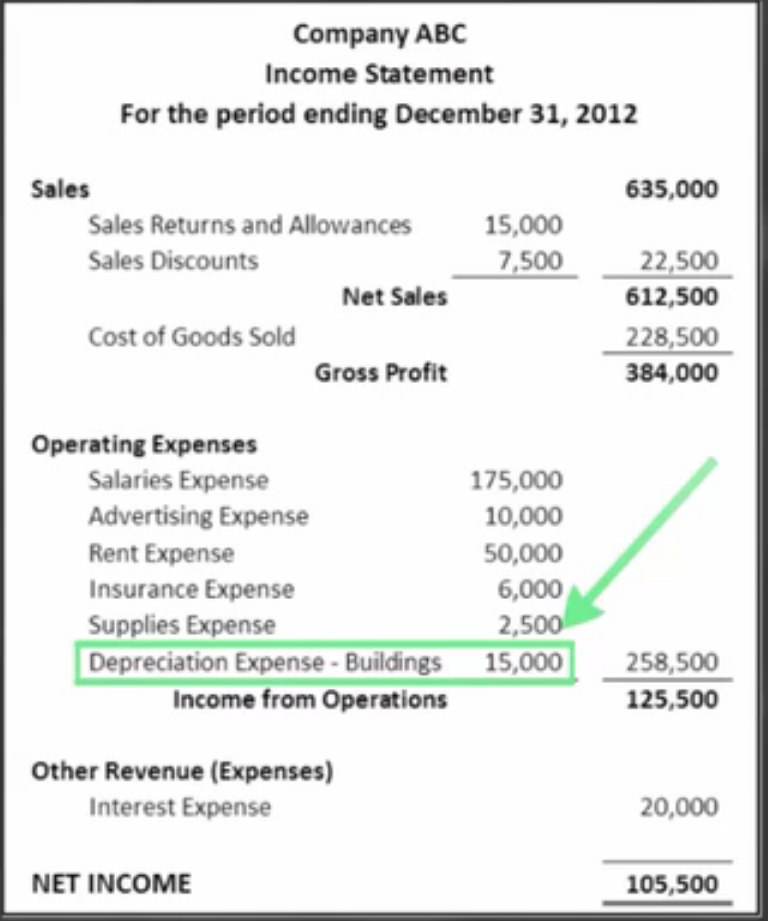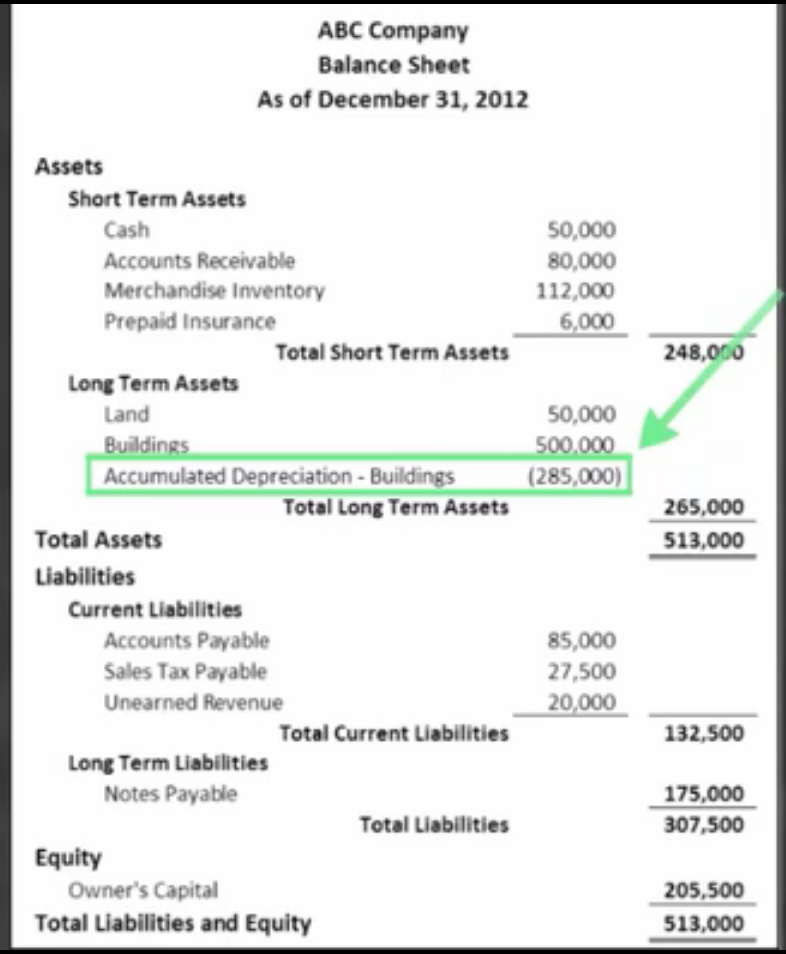# Financial Reporting of Long-Term Assets

Author: Sophia Tutorial
##### Description:

Calculate the book value of a long term asset from an income statement.

(more)### Developing Effective Teams

*No strings attached. This college course is 100% free and is worth 1 semester credit.

37 Sophia partners guarantee credit transfer.

299 Institutions have accepted or given pre-approval for credit transfer.

* The American Council on Education's College Credit Recommendation Service (ACE Credit®) has evaluated and recommended college credit for 32 of Sophia’s online courses. Many different colleges and universities consider ACE CREDIT recommendations in determining the applicability to their course and degree programs.

Tutorial
what's covered
This tutorial will cover financial reporting of long-term assets.

Our discussion breaks down as follows:

1. Reporting Assets and Depreciation
2. Carrying Value
3. Recording Depreciation/Accumulated Depreciation
1. Depreciation Expense
2. Accumulated Depreciation

## 1. Reporting Assets and Depreciation

Assets are reported at their full book value, which is the cost of the depreciable asset less its accumulated depreciation.

We record the asset separately from the accumulated depreciation associated with that asset. Recall that accumulated depreciation is the total depreciation expense that is recorded to date in an asset's life. On the balance sheet, it's reported directly below the related asset.

When reporting, we're going to be reporting fixed assets, also known as capital assets and long-term assets; these terms can be used interchangeably. You may recall that long-term assets are assets that will be used for longer than one year, such as plant assets and long-term investments.

These assets are reported under the "Assets" section of the balance sheet or "Long-Term Assets" section of the balance sheet.

Again, each fixed asset has its own accumulated depreciation line.

## 2. Carrying Value

Carrying value is the book value of an asset, which is cost minus accumulated depreciation. Carrying value is also referred to as book value.

The carrying value includes the cost of the asset and the cost incurred getting it ready for use, such as purchase price, fees, installation costs, etc. The book value also factors in accumulated depreciation, a term you may have noticed in the definition of carrying value: cost less accumulated depreciation.

The formula for calculating carrying value is asset cost, which is the cost of the asset and the cost of getting it ready for use, minus accumulated depreciation.

term to know
Carrying Value
The book value of an asset, cost less accumulated depreciation
formula

Carrying Value## 3. Recording Depreciation/Accumulated Depreciation

Now, let's turn our attention to recording a journal entry related to long-term assets. As a reminder, depreciation is the process of cost allocation to expense of a plant asset over its useful life. It can be calculated using the business's chosen method, whether that is straight line depreciation or accelerated depreciation.

So, what is the journal entry to record depreciation? Well, you would debit "Depreciation Expense" and credit "Accumulated Depreciation" for that specific asset.

3a. Depreciation Expense
Let's go ahead and take a look at where we can find "Depreciation Expense" on an income statement.

Here is a sample income statement for Company ABC, complete with sales, net sales, gross profit, etc.If you focus in on the "Operating Expenses" section of the income statement, you'll see a line item titled "Depreciation Expense," in this case, "Depreciation Expense - Buildings."

Therefore, depreciation expense is reported directly on the income statement as a separate line item.

3b. Accumulated Depreciation
Now, accumulated depreciation is the total depreciation expense recorded to date in an asset's life.

Accumulated depreciation is included on the balance sheet, where we reflect the cost of the asset, as well as the carrying value, which again, is the cost minus the accumulated depreciation.

Here is another example balance sheet. Note, we start with assets, liabilities, and equity.If we look at the "Assets" section, focus in on the "Long-Term Assets" sub-section, and you'll see an "Accumulated Depreciation - Buildings" line item. Right above that line, we have our buildings--a long-term asset which we report at its cost.

Again, directly below that is where we would report the accumulated depreciation for that asset. On a balance sheet, accumulated depreciation is going to be reported directly below the asset that it relates to.

summary
Today we learned all about reporting long-term assets and depreciation. Long-term assets, also known as fixed assets or capital assets, are reported at their carrying value, which is the book value of an asset, expressed as cost less accumulated depreciation. Lastly, we learned about recording depreciation and accumulated depreciation, looking at examples of how depreciation expense shows up on the income statement, as well as how accumulated depreciation is reflected on the balance sheet.

Source: Adapted from Sophia instructor Evan McLaughlin.

Terms to Know
Carrying Value

The book value of an asset, cost less accumulated depreciation.

Rating